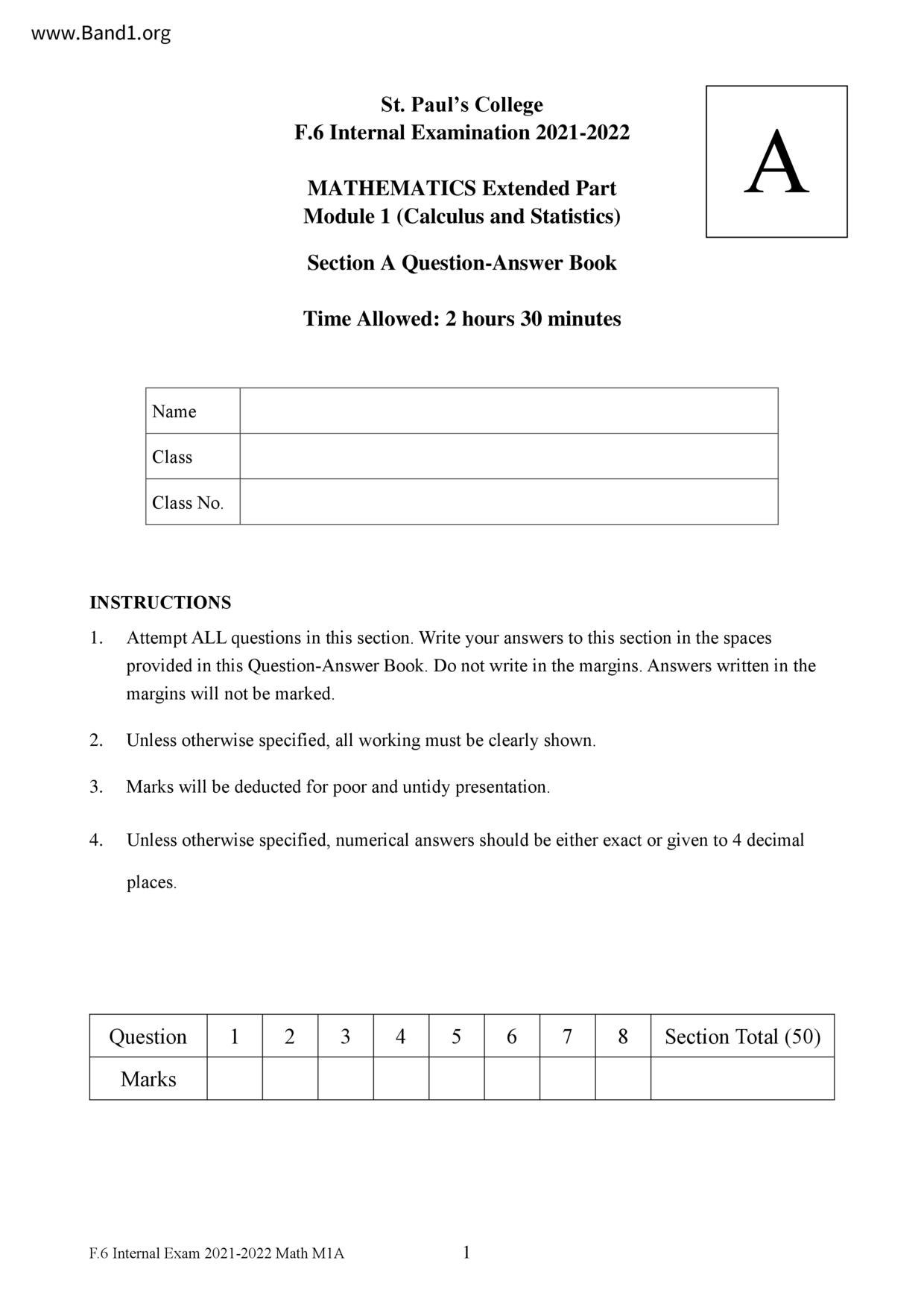# 中六 數學試卷 (F6 Maths Past Paper)

7088

pdf

12

mathematics 2122F6M1SectionAQAB

▼ 圖片只作預覽, 如欲下載整份卷, 請按「免費成為會員」 ▼▲ 圖片只作預覽, 如欲下載整份卷, 請按「免費成為會員」 ▲

## 中六數學試卷 PDF 下載

INSTRUCTIONS
Attempt ALL questions in this section. Write your answers to this section in the spaces
provided in this Question-Answer Book. Do not write in the margins. Answers written in the
margins will not be marked.
St. Paul's College
F.6 Internal Examination 2021-2022
MATHEMATICS Extended Part
Module 1 (Calculus and Statistics)
Time Allowed: 2 hours 30 minutes
Unless otherwise specified, all working must be clearly shown.
Marks will be deducted for poor and untidy presentation.
Unless otherwise specified, numerical answers should be either exact or given to 4 decimal
F.6 Internal Exam 2021-2022 Math MIA
Section Total (50)
Answers written in the margins will not be marked.
Answers written in the margins will not be marked.
F.6 Internal Exam 2021-2022 Math MIA
Answers written in the margins will not be marked.
Answers written in the margins will not be marked.
Let f(x) be a function such that f'(x) =
Answers written in the margins will not be marked.
F.6 Internal Exam 2021-2022 Math M1A
The straight line 6x - 5y -4 = 0 touches the curve y = f(x) at the point A.
It is given that the x-coordinate of A is 2.
where k is a constant.
Answers written in the margins will not be marked.
Answers written in the margins will not be marked.
END OF SECTION A
Answers written in the margins will not be marked.
F.6 Internal Exam 2021-2022 Math M1A
Answers written in the margins will not be marked.
Answers written in the margins will not be marked.
SECTION A (50 marks)
The table below shows the probability distribution of a discrete random variable X, where p
q are constants.
It is given that Var(5X + 1) = 21.
(a) Find p and 9.
(b) Find E(5X + 1).
Answers written in the margins will not be marked.
F.6 Internal Exam 2021-2022 Math M1A
Answers written in the margins will not be marked.
Answers written in the margins will not be marked.
On a particular day, 80% of students in a school take MTR to school and 5% of the students
taking MTR are late to school. It is given that 94% of the students in the school arrive at school
on time. A student from the school is chosen at random.
(a) Find the probability that the chosen student takes MTR to school and arrives on time.
(b) Find the probability that the chosen student does not take MTR and is late to school.
Find the probability that the student arrives school on time given that the chosen student
does not take MTR to school.
Answers written in the margins will not be marked.
F.6 Internal Exam 2021-2022 Math M1A
Answers written in the margins will not be marked.
Answers written in the margins will not be marked.
Let A and B be two events. Denote the complementary event of A by A'. It is given that
and P(B) = P(A' | B).
Show that ² ≤P(A) ≤ ²7.
If A and B are independent, find P(A).
Answers written in the margins will not be marked.
F.6 Internal Exam 2021-2022 Math M1A
Answers written in the margins will not be marked.
Answers written in the margins will not be marked.
A factory manufactures a batch of crystal balls. The diameters of the crystal balls follow a
normal distribution with a mean of 10 cm and a standard deviation of 0.2 cm. A crystal ball is
classified as undersized if its diameter is less than 9.7cm.
(a) Find the probability that a randomly selected crystal ball from the batch is undersized.
(b) 12 crystal balls are randomly selected and packed into a carton. Let X be the random
variable representing the number of undersized crystal balls in the carton. Find
(ii) P(X=2|X<3)
Answers written in the margins will not be marked.
F.6 Internal Exam 2021-2022 Math M1A
Answers written in the margins will not be marked.
Answers written in the margins will not be marked.
Let f(x) = (In x)².
(a) Let g(u) = e" (u² − 2u + 2), where u = ln x. Show that
Answers written in the margins will not be marked.
F.6 Internal Exam 2021-2022 Math M1A
(b) Express, in terms of e, the area of the region bounded by the curve y = f(x), the x-axis and
the straight line x = e.
Answers written in the margins will not be marked.
Answers written in the margins will not be marked.
Answers written in the margins will not be marked.
F.6 Internal Exam 2021-2022 Math MIA
Answers written in the margins will not be marked.
Answers written in the margins will not be marked.
(a) Expand (1-3x²)5 in ascending power of x.
(b) Find the constant k such that the coefficient of x4 in the expansion of ex(1 - 3x²)5
Answers written in the margins will not be marked.
F.6 Internal Exam 2021-2022 Math M1A
Answers written in the margins will not be marked.
Answers written in the margins will not be marked.
(a) Let f(x)=x²-3x² - 9x +32 for 0≤x≤10.
Show that f(x) > 0 for 0≤x≤10.
(b) Let g(x) = ln (x³ - 3x² - 9x +32) for 0≤x≤10.
Find the greatest value of g(x) for 0≤x≤10.
Answers written in the margins will not be marked.
F.6 Internal Exam 2021-2022 Math M1A
Answers written in the margins will not be marked.